GFG App
Open AppBrowser
Continue

Triangles are three-sided polygon which have three vertices. Basic construction techniques allow us to construct triangles. An important property of the triangle is that sum of internal angles of a triangle is 180°.  SAS, SSS, ASA, and RHS are the rules of congruency of two triangles. A triangle is unique if:

1. It’s two sides and the angle included between them is given.
2. Three sides are given or two angles and an included side is given.
3. Three sides are given.
4. The hypotenuse and one side are given for a right-angled triangle.

Let’s look at all of these techniques to construct the sides of a triangle.

### Construct a triangle, given its base, a base angle, and sum of the other two sides

Our goal is to construct a triangle with given base AB, a base angle ∠B, and the sum of the other two sides AC + BC.

Steps for Construction:

Step 1. Draw line segment AB and at the end of the line segment at point A. Construct an angle ∠MAB which is equal to the given angle.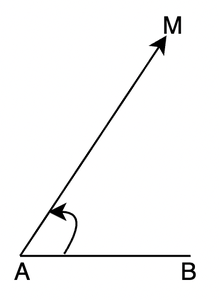Step 2. Cut the line segment AD equal to AC + BC from ray AM.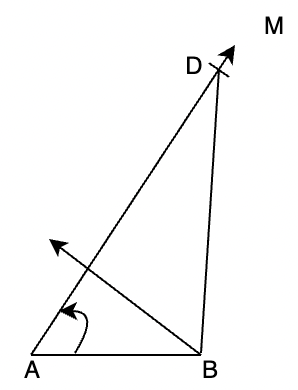Step 3. Join BM and make an angle DBC equal to ∠BDC.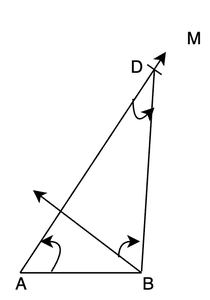Step 4. Let CY intersect BX at C.

Thus, ABC is a required triangle. In the triangle we can see, Base BC and ∠B are drawn as per the given requirement. In triangle BCD,

∠CDB = ∠CBD (By construction)

Therefore, BC = CD and then,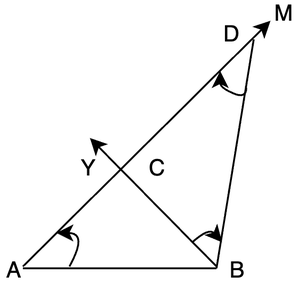### Construction of a Scalene triangle, given its perimeter and two base angles

In this construction, our goal is to construct a triangle given the base angles Q and R and the perimeter(PQ + QR + PR).

Steps for Construction:

Step 1. Draw a line segment XY whose length is equal to the perimeter PQ + QR + PR.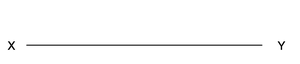Step 2. Construct angles LXY equal to ∠Q and angle MYX equal to ∠R.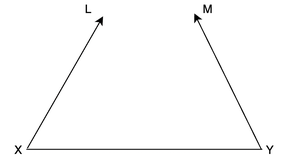Step 3. Draw bisectors of these angles ∠LXY and ∠MYX. Extend these bisectors to make them intersect at P.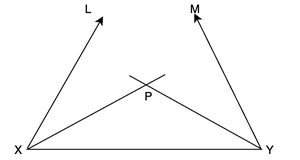Step 4. Draw perpendicular bisectors AB of PX and CD of PY.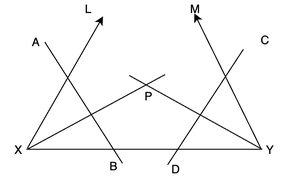Step 5. Extend AB to intersect XY at Q and CD to intersect XY at C. Join the line segments PQ and PR.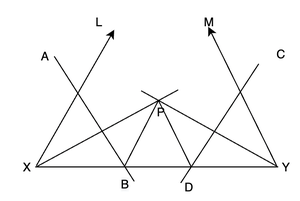So, PQR is the required triangle. We can verify it in the following way,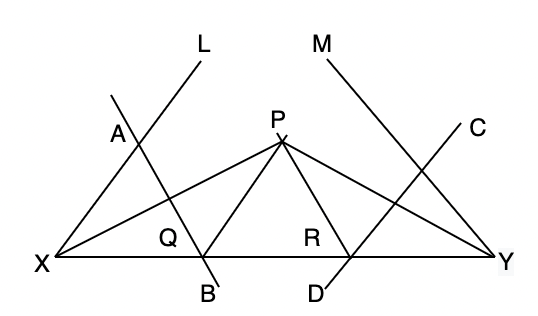### Construct a triangle given its base, a base angle, and the difference of the other two sides

The goal is to construct a triangle ABC when base BC, base angle B, and the difference of the other two sides AB – AC or AC – AB is given. With this description, there are two possible cases:

Case (i): AB – AC is given, that is AB > AC.

Case (ii): AC – AB is given, that is AC > AB.

Let’s look at both of the cases,

Case (i): PQ – PR is given, that is PQ > PR

Step 1. We need to draw the base first, Draw line segment QR, and at point Q make an angle XQR equal to the given angle.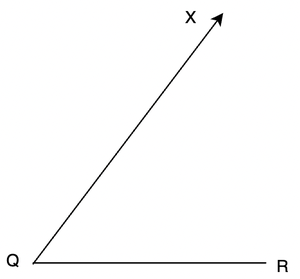Step 2. Cut the line segment QS equal to PQ – PR from ray QX.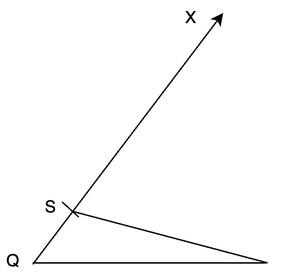Step 3. Join RS and draw a perpendicular bisector of RS.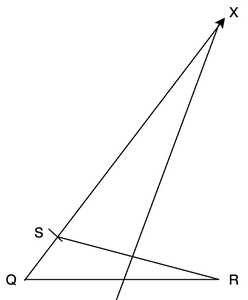Step 4. Let it intersect QX at point P. Join PR.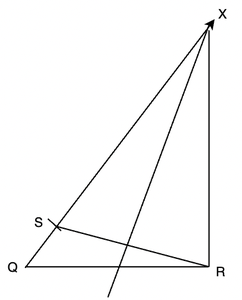Let’s verify how the triangle obtained is the required triangle ABC.

The base of the triangle, QR, and angle Q are drawn equal to what is given. Notice that point P is lying on the perpendicular bisector of SR. So,

PS = PR,

QS = PQ – PS = PQ – PR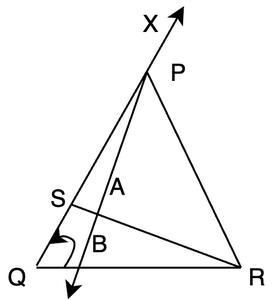Case (ii): Let PQ < PR that is PR – PQ is given

Step 1. This step is similar to the previous case.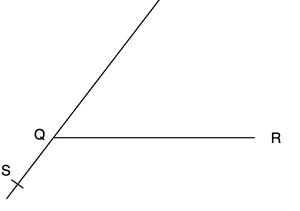Step 2. Cut a line segment QS whose length is equal to PR – PQ from the line QX extended on the opposite side of line segment QR.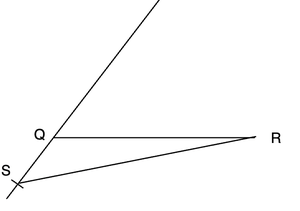Step 3. Join SR and draw the perpendicular bisector, say PT of RQ.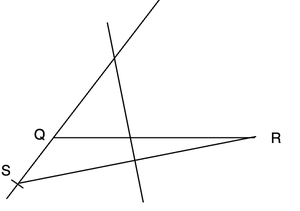Step 4. Let PT intersect QX at P. Join PR.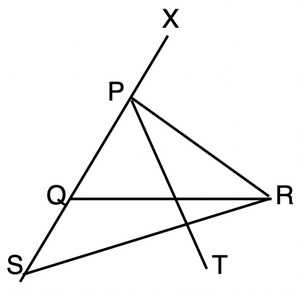Then, QPR is the required triangle.

The verification of this construction can also be done in the same way as the Case (i).

### Sample Problems

Question 1: Construct a Triangle with sides XY= 8cm, YZ= 10cm, XZ= 12cm.

Solution:

Steps:

Step 1. Draw YZ=10cm.

Step 2. From Y, measure 12cm from a compass and draw an arc.

Step 3. From Z, measure 8cm from a compass and draw another arc.

Step 4. The point where both arcs intersect each other is point X.

Step 5. Join X to Y and X to Z.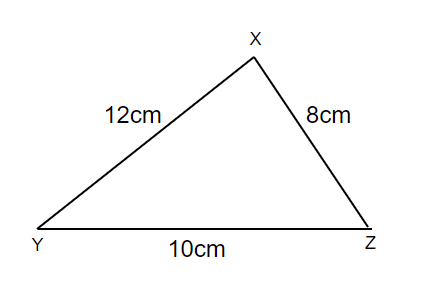Question 2: A triangle PQR can be constructed in which PQ = 5 cm, ∠P = 45° and QR + PR = 5 cm. State whether it is True or False.

This statement is False, because in triangles sum of two sides is greater than the other side.

So, QR + PR should be greater than PQ. But it is not the case, in fact they are equal. Thus, this statement is False.

Question 3: Which of the following angles can be constructed with the help of a compass.

1. 35°
2. 40°
3. 37.5°
4. 47.5°

37.5°

If we make a 150° angle and bisect it, We will get 75° and then on further bisecting it, we will get 37.5°.

Question 4: Construct a Triangle ABC having ∠A= 60°, ∠B= 60°. Also, AB+ BC+ AC= 12cm.

Steps:

Step 1. Draw a line XY of 12cm

Step 2. From X, make an angle of 60°

Step 3. From Y, draw an angle 60°.

Step 4. Make angle bisectors of both the angles, the line will meet at C.

Step 5. Draw the perpendicular bisector of line XC and YC

Step 6. The Perpendicular bisectors will meet the line XY at A and B.

Step 7. We have obtained our Triangle with ∠A= 60°, ∠B= 60°, and AB+BC+CA= 12cm.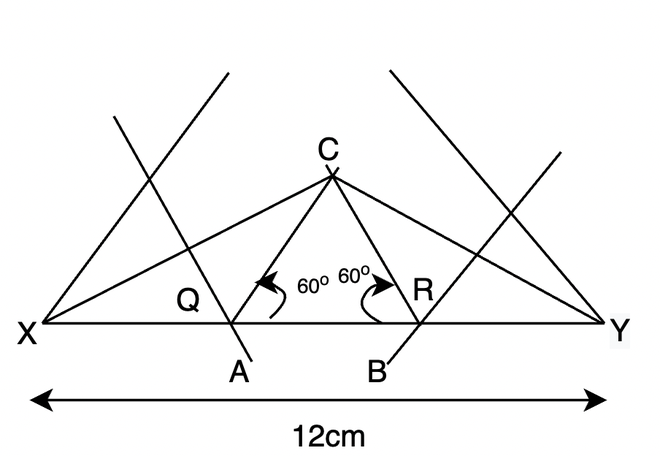Question 5: Construct a Triangle PQR, where ∠P=60°, ∠Q= 45°, PQ= 10cm.

Solution:

Steps:

Step 1. Draw a line PQ=10cm

Step 2. From point P, Make an angle of 60°

Step 3. From point Q, make an angle of 45°

Step 4. The point where both angles’ arcs intersect each other is point R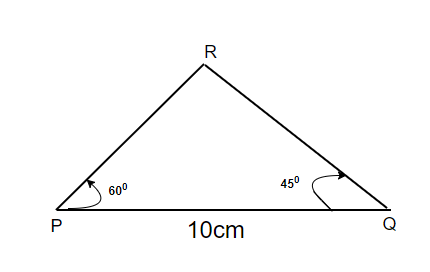My Personal Notes arrow_drop_up